A block of mass m = 25 kg on a smooth horizontal surface with a velocity $\stackrel{⇀}{v}$ = 3$m{s}^{-1}$ meets the spring of spring constant k = 100 N/m fixed at one end as shown in the figure. The maximum compression of the spring and velocity of the block as it returns to the original position respectively are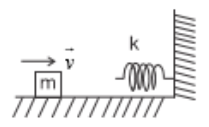1.  1.5m, -3$m{s}^{-1}$

2.  1.5m, 0$m{s}^{-1}$

3.  1.0m, 3$m{s}^{-1}$

4.  0.5m, 2$m{s}^{-1}$

Concept Questions :-

Elastic potential energy
High Yielding Test Series + Question Bank - NEET 2020

Difficulty Level:

The velocity, given to the block of mass (m), is to rotate it in a circle of radius l. Calculate the height (h) where the block leaves the circle.

(1) $\frac{3l}{2}$

(2) $\frac{4l}{3}$

(3) $\frac{5l}{4}$

(4) None of these

Concept Questions :-

Work-Energy theorem
High Yielding Test Series + Question Bank - NEET 2020

Difficulty Level: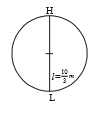where = maximum tension on the string

1.  10 m/s

2.  20 m/s

3.  $\frac{10}{\sqrt{2}}\mathrm{m}/\mathrm{s}$

4.

Concept Questions :-

Work-Energy theorem
High Yielding Test Series + Question Bank - NEET 2020

Difficulty Level:

The relation between velocity (v) and time (t) is $\mathrm{v\alpha }\sqrt{\mathrm{t}}$, then which one of the following quantity is constant:

1.  Force

2.  Power

3.  Momentum

4.  Kinetic Energy

Concept Questions :-

Power
High Yielding Test Series + Question Bank - NEET 2020

Difficulty Level:

A particle is moving on the circular path of the radius (R) with centripetal acceleration ${a}_{c}={k}^{2}R{t}^{2}$. Then the correct relation showing power (P) delivered by net force versus time (t) is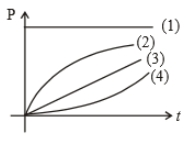1. 1

2. 2

3. 3

4. 4

Concept Questions :-

Power
High Yielding Test Series + Question Bank - NEET 2020

Difficulty Level:

A steel wire can withstand a load up to 2940 N. A load of 150 kg is suspended from a rigid support. The maximum angle through which the wire can be displaced from the mean position, so that the wire does not break when the load passes through the position of equilibrium, is (2008 E)

(1) 30$°$

(2) 60$°$

(3) 80$°$

(4) 85$°$

Concept Questions :-

Work-Energy theorem
High Yielding Test Series + Question Bank - NEET 2020

Difficulty Level:

A body is thrown vertically up with certain initial velocity, the potential and kinetic energies of the body are equal at a point P in its path. If the same body is thrown with double the velocity upwards, the ratio of potential and kinetic energies of the body when it crosses the same point, is

1. 1:1

2. 1:4

3. 1:7

4. 1:8

Concept Questions :-

Gravitational Potential energy
High Yielding Test Series + Question Bank - NEET 2020

Difficulty Level:

A body is displaced from (0,0) to (1m,1m) along the path x=y by a force $F=\left({x}^{2}\stackrel{^}{j}+y\stackrel{^}{i}\right)N$. The work done by this force will be :

1. $\frac{4}{3}J$

2. $\frac{5}{6}J$

3. $\frac{3}{2}J$

4. $\frac{7}{5}J$

Concept Questions :-

Work done by variable force
High Yielding Test Series + Question Bank - NEET 2020

Difficulty Level:

In the figure shown, the potential energy U of a particle is plotted against its position 'x' from the origin. Then which of the following statement is correct?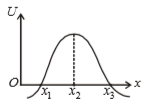1. ${\mathrm{x}}_{1}$ is in stable equilibrium

2. ${\mathrm{x}}_{2}$ is in stable equilibrium

3. ${\mathrm{x}}_{3}$ is in stable equilibrium

4. none of these

Concept Questions :-

Potential energy: Relation with force
High Yielding Test Series + Question Bank - NEET 2020

Difficulty Level:

A weightless rod of length 2l carries two equal mass 'm', one tied at lower end A and the other at the middle of the rod at B. The rod can rotate in a vertical plane about a fixed horizontal axis passing through C. The rod is released from rest in the horizontal position. The speed of the mass B at the instant rod, become vertical is: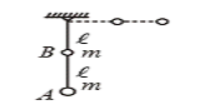(1) $\sqrt{\frac{3gl}{5}}$

(2) $\sqrt{\frac{4gl}{5}}$

(3) $\sqrt{\frac{6gl}{5}}$

(4) $\sqrt{\frac{7gl}{5}}$

Concept Questions :-

Work-Energy theorem